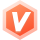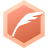# 变量的赋值与表达式迂者-贺利坚烟台大学计算机学院教师博客专家认证
2023-01-12 13:51:31

...全文
3 回复 打赏 收藏 举报C语言程序设计-赋值运算符与赋值表达式.pptx扩展赋值运算符
1.= 赋值运算符 变量=表达式 如：a=3；将a的值赋为3 2./= 除后赋值 变量/=表达式 如：a/=3;即a=a/3 3.*= 乘后赋值 变量*=表达式 如：a*=3;即a=a*3 4.%= 取模后赋值 变量%=表达式 如：a%=3;即a=a%3 5.+= 加后赋值 变量+=表达式 如：a+=3;即a=a+3 6.-= 减后赋值 变量-=表达式赋值运算符C语言 赋值表达式在C语言中11种赋值运算符的具体含义，用法
1.= 赋值运算符 变量=表达式 如：a=3；将a的值赋为3 2./= 除后赋值 变量/=表达式 如：a/=3;即a=a/3 3.*= 乘后赋值 变量*=表达式 如：a*=3;即a=a*3 4.%= 取模后赋值 变量%=表达式 如：a%=3;即a=a%3 5.+= 加后赋值 变量+=表达式 如：a+=3;即a=a+3 6.-= 减后赋值 变量-=表达式

1